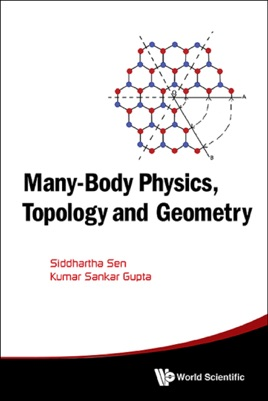• 28,99 €

## Description de l’éditeur

The book explains concepts and ideas of mathematics and physics that are relevant for advanced students and researchers of condensed matter physics. With this aim, a brief intuitive introduction to many-body theory is given as a powerful qualitative tool for understanding complex systems. The important emergent concept of a quasiparticle is then introduced as a way to reduce a many-body problem to a single particle quantum problem. Examples of quasiparticles in graphene, superconductors, superfluids and in a topological insulator on a superconductor are discussed.

The mathematical idea of self-adjoint extension, which allows short distance information to be included in an effective long distance theory through boundary conditions, is introduced through simple examples and then applied extensively to analyse and predict new physical consequences for graphene.

The mathematical discipline of topology is introduced in an intuitive way and is then combined with the methods of differential geometry to show how the emergence of gapless states can be understood. Practical ways of carrying out topological calculations are described.
Contents:OverviewMany-Body TheoryTopology and GeometryBoundary Conditions and Self-Adjoint ExtensionsElectronic Properties of Graphene
Readership: Graduate students and researchers in condensed matter physics and mathematical physics.

Key Features:Topics are of current interest, e.g. graphene, topological insulators, Majorana fermionsIs self-contained and provides all the background material necessary to understand the physical or mathematical concepts discussedPractical ways of using topology, self-adjoint extensions as well as ways of making qualitative estimates in physics are explained and then illustrated by examples